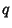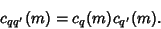## Ramanujan's Sum

The sum(1)

whereruns through the residues Relatively Prime to, which is important in the representation of numbers by the sums of squares. If(i.e.,and' are Relatively Prime), then(2)

For argument 1,(3)

whereis the Möbius Function, and for general,(4)